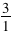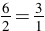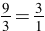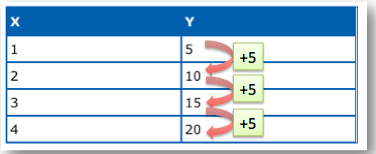# Direct Variation

Each day we come across many situations in science, engineering, industry, and daily life where the value of one quantity depends upon the quantity of another. The individuals who find these situations are in search of the relationship between the quantities. This relationship can be expressed as formulas or equations.

When these two quantities have a constant (unchanged) ratio, their relationship is known as direct variation. Many individuals say that one variable “varies directly” as the other. The constant ratio is called the constant of variation.

Let’s look at it this way.

You know that there are 3 feet in 1 yard, 6 feet in 2 yards, 9 feet in 3 yards.   As the number of feet increases, the number of yards increases.

 Feet 3 6 9 Yards 1 2 3Each ratio of feet to yards is equivalent to 3:1.   The relationship between feet and yards varies directly.

The relationships between the changing quantities can be examined by using a model for data that illustrates how the change in one variable affects a second variable.

A direct variation relationship can be represented by a linear equation in the form y = kx, where k is called the constant of proportionality (or constant of variation).

## The Formula

y = kx ,

where k is the constant of variation (constant ratio).
“y varies directly as x”

## In a Table

X Y
1 4
2 8
3 12
4 16

From the table we can gather the information necessary to create the formula for direct variation which indicates actual variation. We notice that the variable y is always 4 times the variable x. This can be seen through the table on each row. Thus, our constant is k or 4.

Our formula or equation would be y = 4x.

## Real Life

Real life Instance of quantities that rely on one another :

A grocery store cashier who is paid hourly knows that working longer means making more money. That’s because his pay varies directly with the number of hours worked. As his hours increase, so does the amount of his paycheck. A race car driver knows that completing 100 laps before making a pit stop is better than completing only 80, because distance is directly proportional to time when driving at a constant speed. The longer she drives, the more distance she’ll cover.

### Example 1

What is the relationship between x and y in the table?

As the x value increases, the y values increase by 5.   This is representative of direct variation.### Example 2

What would our direct variation equation be if the constant of variation is 10?

Since the direct variation formula is y = kx and the k represents the constant of variation, the equation would be y = 10x.

(source)